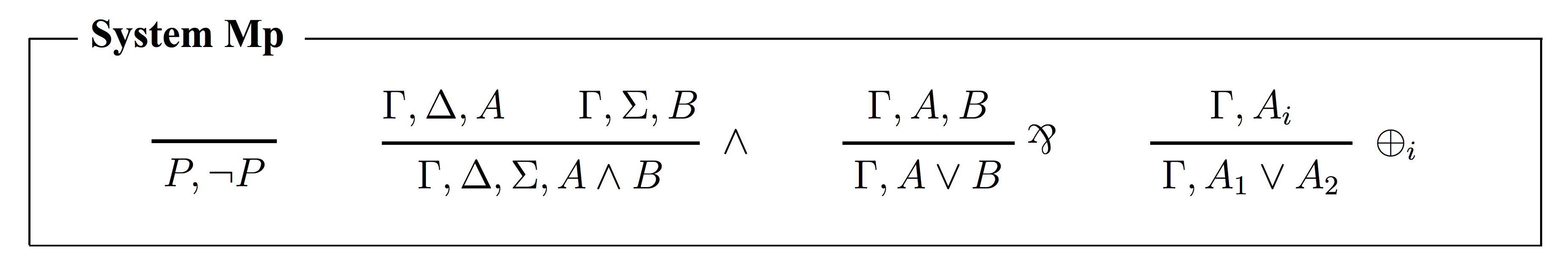### Dominic Hughes - Selected Papers

A classical sequent calculus free of structural rules
Annals of Pure and Applied Logic, Volume 161, Issue 10, July 2010, Pages 1244-1253.
Archived as math.LO/0506463 at arXiv.org. [bibtex]

Gentzen's classical sequent calculus LK has explicit structural rules for contraction and weakening. They can be absorbed (in a right-sided formulation) by replacing the axiom P, ¬P by Γ,P,¬P for any context Γ, and replacing the original disjuction rule with Γ,A,B implies Γ,A∨B.

This paper presents a classical sequent calculus which is also free of contraction and weakening, but more symmetrically: both contraction and weakening are absorbed into conjunction, leaving the axiom rule intact. It uses a blended conjunction rule, combining the standard context-sharing and context-splitting rules: Γ,Δ,A and Γ,Σ,B implies Γ,Δ,Σ,A∧B. We refer to this system M as minimal sequent calculus.

We prove a minimality theorem for the propositional fragment Mp: any sequent calculus S (within a standard class of right-sided calculi) is complete if and only if S contains Mp (that is, each rule of Mp is derivable in S). Thus one can view Mp as a minimal complete core of Gentzen's LK.

Keywords: Proof theory; Sequent calculus; Gentzen; Structural rules

Mathematical subject codes: 03B05; 03B20; 03B47